## Quartic Equation

A general quartic equation (also called a Biquadratic Equation) is a fourth-order Polynomial of the form(1)

The Roots of this equation satisfy Newton's Relations: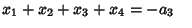(2)(3)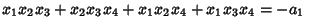(4)(5)
where the denominators on the right side are all.

Ferrari was the first to develop an algebraic technique for solving the general quartic. He applied his technique (which was stolen and published by Cardano) to the equation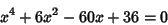(6)

(Smith 1994, p. 207).

Theterm can be eliminated from the general quartic (1) by making a substitution of the form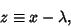(7)

so(8)
Lettingso(9)

then gives(10)

where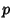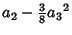(11)(12)(13)

Adding and subtractingto (10) gives(14)

which can be rewritten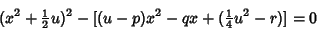(15)

(Birkhoff and Mac Lane 1965). The first term is a perfect square, and the second term is a perfect square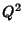for thosesuch that(16)

This is the resolvent Cubic, and plugging a solutionback in gives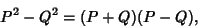(17)

so (15) becomes(18)

where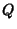(19)(20)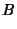(21)

Letbe a Real Root of the resolvent Cubic Equation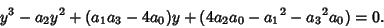(22)

The four Roots are then given by the Roots of the equation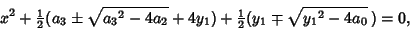(23)

which are(24)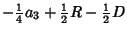(25)(26)(27)

where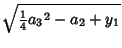(28)(29)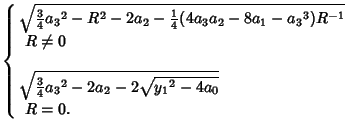(30)

Another approach to solving the quartic (10) defines(31)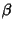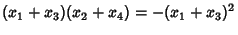(32)(33)

where use has been made of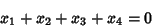(34)

(which follows since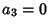), and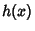(35)(36)

Comparing with(37)(38)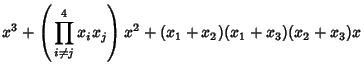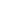(39)

gives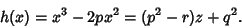(40)

Solving this Cubic Equation gives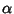,, and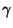, which can then be solved for the roots of the quartic(Faucette 1996).

References

Abramowitz, M. and Stegun, C. A. (Eds.). Handbook of Mathematical Functions with Formulas, Graphs, and Mathematical Tables, 9th printing. New York: Dover, pp. 17-18, 1972.

Berger, M. §16.4.1-16.4.11.1 in Geometry I. New York: Springer-Verlag, 1987.

Beyer, W. H. CRC Standard Mathematical Tables, 28th ed. Boca Raton, FL: CRC Press, p. 12, 1987.

Birkhoff, G. and Mac Lane, S. A Survey of Modern Algebra, 3rd ed. New York: Macmillan, pp. 107-108, 1965.

Ehrlich, G. §4.16 in Fundamental Concepts of Abstract Algebra. Boston, MA: PWS-Kent, 1991.

Faucette, W. M. A Geometric Interpretation of the Solution of the General Quartic Polynomial.'' Amer. Math. Monthly 103, 51-57, 1996.

Smith, D. E. A Source Book in Mathematics. New York: Dover, 1994.

van der Waerden, B. L. §64 in Algebra, Vol. 1. New York: Springer-Verlag, 1993.